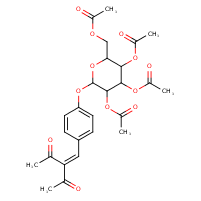Structure InformationCompound Identification

SMILES

CC(=O)OCC1OC(OC2=CC=C(C=C(C(C)=O)C(C)=O)C=C2)C(OC(C)=O)C(OC(C)=O)C1OC(C)=O

InChIKey

InChIKey=BPTAOHLPWUOFGS-UHFFFAOYSA-N

Formula

C26H30O12

Mass

534.514